# HSSlive: Plus One & Plus Two Notes & Solutions for Kerala State Board

## AP Board Class 7 Maths Chapter 4 Lines and Angles InText Questions Textbook Solutions PDF: Download Andhra Pradesh Board STD 7th Maths Chapter 4 Lines and Angles InText Questions Book AnswersAP Board Class 7 Maths Chapter 4 Lines and Angles InText Questions Textbook Solutions PDF: Download Andhra Pradesh Board STD 7th Maths Chapter 4 Lines and Angles InText Questions Book Answers

## Andhra Pradesh State Board Class 7th Maths Chapter 4 Lines and Angles InText Questions Books Solutions

 Board AP Board Materials Textbook Solutions/Guide Format DOC/PDF Class 7th Subject Maths Chapters Maths Chapter 4 Lines and Angles InText Questions Provider Hsslive

2. Click on the Andhra Pradesh Board Class 7th Maths Chapter 4 Lines and Angles InText Questions Answers.
3. Look for your Andhra Pradesh Board STD 7th Maths Chapter 4 Lines and Angles InText Questions Textbooks PDF.
4. Now download or read the Andhra Pradesh Board Class 7th Maths Chapter 4 Lines and Angles InText Questions Textbook Solutions for PDF Free.

## AP Board Class 7th Maths Chapter 4 Lines and Angles InText Questions Textbooks Solutions with Answer PDF Download

Find below the list of all AP Board Class 7th Maths Chapter 4 Lines and Angles InText Questions Textbook Solutions for PDF’s for you to download and prepare for the upcoming exams:

Try This

Question 1.
How many transversals can be drawn for two distinct lines? (Page No.77)
Solution:
Infinite number of transversals can be drawn for two distinct lines.

DoThis:

Question 1.
Identify the transversal in figure (1) and (ii). Identify the exterior and interior angles and fill the table given below. (Page No. 78)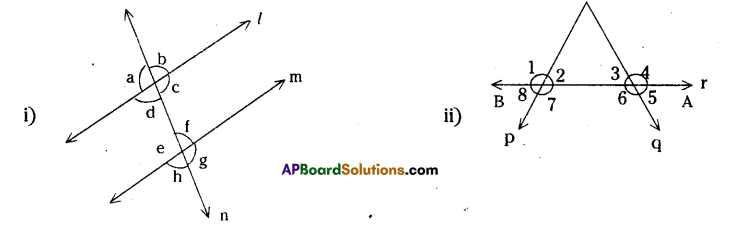Solution:

 Figure Transversal Exterior angles Interior angles i) n ∠a, ∠b, ∠g, ∠h ∠c, ∠d, ∠e, ∠f ii) r ∠1, ∠4, ∠5, ∠8 ∠2, ∠3, ∠6, ∠7

Question 2.
Consider the following lines. Which line ¡s a transversal. Number and list all the angles formed. Which are the exterior angles and which are the interior angles (Page No.79)
Solution: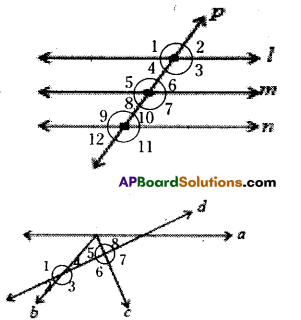For fig. (i) P is transversal for l, m and n.
∠3, ∠4, ∠5, ∠6 are interior angles for l, m.
∠7, ∠8, ∠9, ∠10 are Interior angles for m, n.
∠1, ∠2, ∠7, ∠8 are exterior angles for l, m.
∠1, ∠2, ∠11, ∠12 are exterior angles for l, n.
for fig (ii),
line d is transversal for pair of lines b and c.
∠3, ∠4, ∠5, ∠6 are interior angles.
∠1, ∠2, ∠7, ∠8 are exterior angles.

Do This

Question 1.
Name the pairs of angles in each figure by their property. (Page no. 80)Question 2.
Fill the table with the measures of the corresponding angles. (Page no. 83)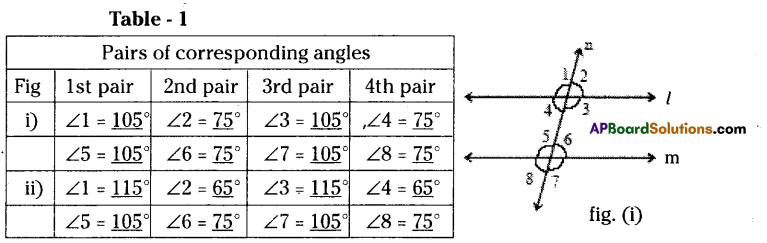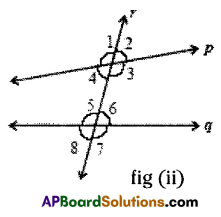1. Find out in which figure the pairs of corresponding angles are equal
In fig. (I) pairs of corresponding angles are equal. q

2. What can you say about the lines ‘1’ and ‘m’?
l is parallel to m, fig (ii)

3. What can you say about the lines ‘p’ and ‘q’?
In fig. (ii) pairs of corresponding angles are not equal and hence p is not parallel to q.

4. Which pair of lines are parallel?
L and M are parallel.

Fill the table with the measures of the interior alternate angles. (Page no. 84)

Table – 2

 Fig Pairs of interior alternate angles 1st pair 2nd pair i) ∠3 = 105° ∠4 = 75° ∠5 = 105° ∠6 = 75° ii) ∠3 = 115° ∠4 = 65° ∠5 = 105° ∠6 = 75°

1. Find out in which the pair of interior ¿ ternate angles are equal?
In fig (i) the pairs of alternate interior angles are equal.

2. What can you say about lines ‘L’ and ‘M’?
L||m.

3. What can you say about the lines ‘p’ and ‘q’?
In fig (ii) the pair of alternate interior angles are not equal. Therefore p ∦ q

Try This

Question 1.
Write any five pairs of supplementary angles of your choice. (Page no 72)
Solution:
(80°, 100°), (60°, 120°), (108°, 72°) (140°, 40°). 30°, 150°)

Try This

Question 1.
Draw five pairs of complementary angles of your choice. (Page no. 71)
Solution:
The following are the pairs of complementary angles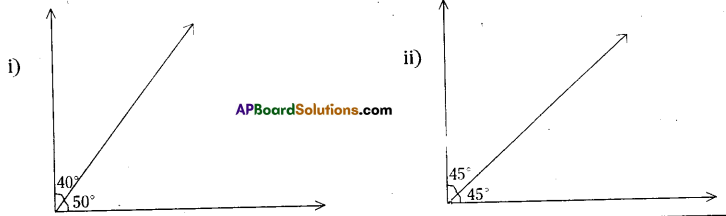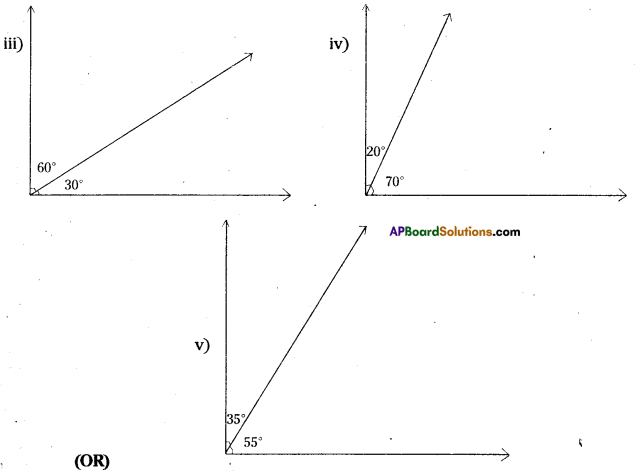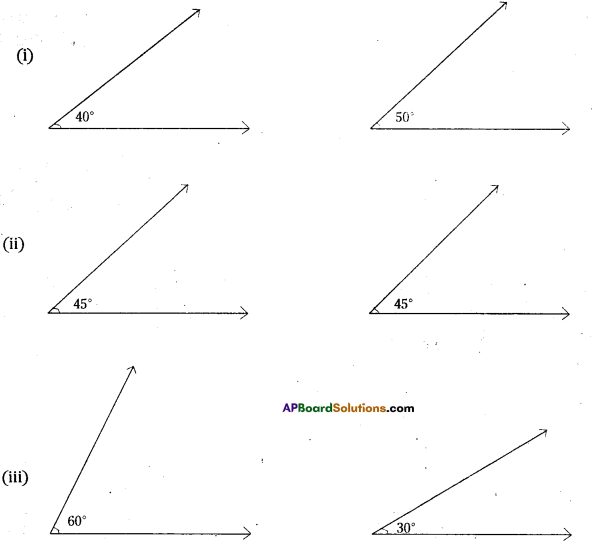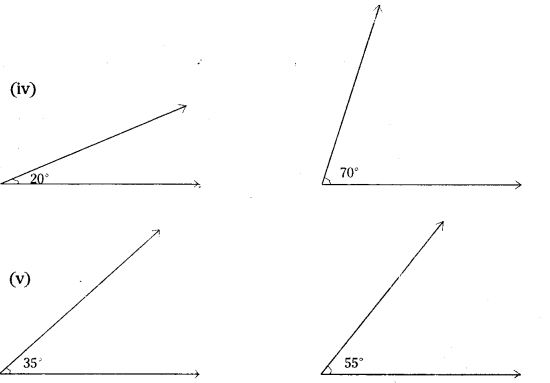Do This

Question 1.
Draw an angle ∠AOB = 40°. With the same vertex 0’ draw ∠BOC = 50°, taking 𝐎𝐁−→− Initial ray as shown In the figure. Since the sum of these angles Is 90°, they together form a right angle. Take another pair 60° and 50° and join in the same way. Do they form complementary angles? Why? Why not? (Page No. 71)
Solution:
∠AOB = 40°, ∠BOC = 50°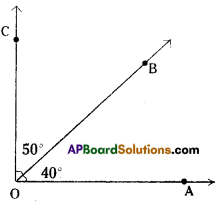∠POQ = 60°
∠QOR = 50°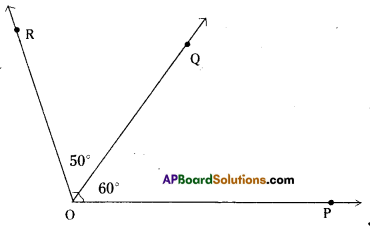These two do not form pair of complementary angles since their sum is 60° + 50° = 110° ≠ 90°

Question 2.
Draw an angle ∠AOB = 100° with the same vertex O, draw ∠BOC = 80° such that OB⎯⎯⎯⎯⎯⎯⎯⎯ is common to two angles. (Page No. 72)
Solution:
∠AOB = 100°
∠BOC = 80°
∠AOB +∠BOC = 180°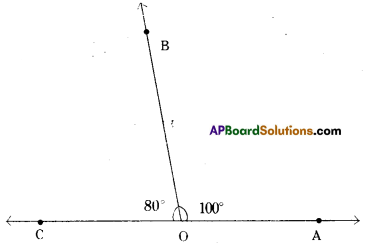Question 3.
Are 130° and 70° supplementary angles? Why? Why not?
∠POQ = 130°, ∠QOR = 70°
∠POQ + ∠QOR = 130° + 70° = 200° ≠ 180°
Hence they are not supplementary angles.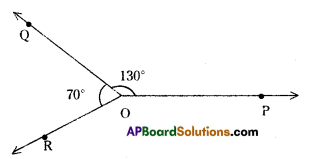## Andhra Pradesh Board Class 7th Maths Chapter 4 Lines and Angles InText Questions Textbooks for Exam Preparations

Andhra Pradesh Board Class 7th Maths Chapter 4 Lines and Angles InText Questions Textbook Solutions can be of great help in your Andhra Pradesh Board Class 7th Maths Chapter 4 Lines and Angles InText Questions exam preparation. The AP Board STD 7th Maths Chapter 4 Lines and Angles InText Questions Textbooks study material, used with the English medium textbooks, can help you complete the entire Class 7th Maths Chapter 4 Lines and Angles InText Questions Books State Board syllabus with maximum efficiency.

## FAQs Regarding Andhra Pradesh Board Class 7th Maths Chapter 4 Lines and Angles InText Questions Textbook Solutions

#### Can we get a Andhra Pradesh State Board Book PDF for all Classes?

Yes you can get Andhra Pradesh Board Text Book PDF for all classes using the links provided in the above article.

## Important Terms

Andhra Pradesh Board Class 7th Maths Chapter 4 Lines and Angles InText Questions, AP Board Class 7th Maths Chapter 4 Lines and Angles InText Questions Textbooks, Andhra Pradesh State Board Class 7th Maths Chapter 4 Lines and Angles InText Questions, Andhra Pradesh State Board Class 7th Maths Chapter 4 Lines and Angles InText Questions Textbook solutions, AP Board Class 7th Maths Chapter 4 Lines and Angles InText Questions Textbooks Solutions, Andhra Pradesh Board STD 7th Maths Chapter 4 Lines and Angles InText Questions, AP Board STD 7th Maths Chapter 4 Lines and Angles InText Questions Textbooks, Andhra Pradesh State Board STD 7th Maths Chapter 4 Lines and Angles InText Questions, Andhra Pradesh State Board STD 7th Maths Chapter 4 Lines and Angles InText Questions Textbook solutions, AP Board STD 7th Maths Chapter 4 Lines and Angles InText Questions Textbooks Solutions,
Share: